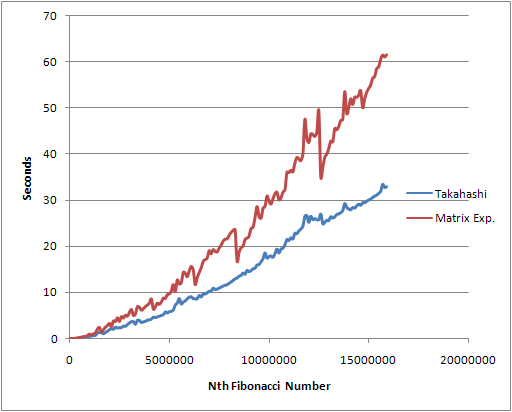9. Algorithms
 Previous Index Next
Fast Fibonacci Numbers

One of the common methods for calculating fibonacci numbers quickly involves matrix exponentiation. I recently came across a new algorithm for generating Fibonacci numbers by Japanese mathematician Takahashi Daisuke. Here is my implementation:

```from math import log, floor
def fib (n):
if n == 0:
return n
F, L, sign, exp = 1, 1, -2, int(floor(log (n,2)))
for i in xrange (exp - 1):
F2   = F**2
FL2  = (F + L)**2
F    = ((FL2 - 6*F2) >> 1) - sign
if n & mask:
temp = (FL2 >> 2 ) + F2
L     = temp + (F << 1)
F     = temp
else:
L    = 5*F2 + sign
sign = -2 if n & mask else 2
if n & (mask >> 1) == 0:
return F * L
else:
return ((F + L) >> 1) * L - (sign >> 1)
```

Here is a comparison of my implementation of the Daisuke algorithm above versus an implementation of the matrix exponentiation algorithm (source unfortunately can't be found right now):About the author I'm Steve Krenzel, a software engineer and co-founder of Thinkfuse. Contact me at steve@thinkfuse.com.Previous Next